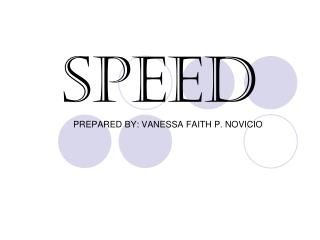DownloadDownload PresentationSPEED

# SPEED

Download Presentation## SPEED

- - - - - - - - - - - - - - - - - - - - - - - - - - - E N D - - - - - - - - - - - - - - - - - - - - - - - - - - -
##### Presentation Transcript

1. SPEED PREPARED BY: VANESSA FAITH P. NOVICIO

2. INSTRUCTIONS • Read the questions carefully. • Choose your answer. • After answering, click the “next question” at the upper-right part and the next question will appear. • Do the same after.

3. Next question Question #1 What is the speed of the boy if in 2 hours, he is already 4 kilometers away from his house? a.20 km/h c.2 km/h b.3 km/h d.12 km/h

4. Next question What is the speed of the boy if in 2 hours, he is already 4 kilometers away from his house? a.20 km/h c.2 km/h b.3 km/h d.12 km/h You’re answer is correct!

5. Next question What is the speed of the boy if in 2 hours, he is already 4 kilometers away from his house? a.20 km/h c.2 km/h b.3 km/h d.12 km/h You’re answer is wrong! The correct answer is. . .

6. Next question Question #2 The average speed of a car for the first 5 hours is 90 km/h. Its average speed for the next 4 hours is 80 km/h. Find its average speed for the whole trip. a.470 km/h c.772 km/h b.770 km/h d.707 km/h

7. Next question The average speed of a car for the first 5 hours is 90 km/h. Its average speed for the next 4 hours is 80 km/h. Find its average speed for the whole trip. a.470 km/h c.772 km/h b.770 km/h d.707 km/h You’re answer is correct!

8. Next question The average speed of a car for the first 5 hours is 90 km/h. Its average speed for the next 4 hours is 80 km/h. Find its average speed for the whole trip. a.470 km/h c.772 km/h b.770 km/h d.707 km/h You’re answer is wrong! The correct answer is. . .

9. Next question Question #3 What do you call the speed that is unchanged during a period of time? a. Uniform Speed c. Same Speed b. Average Speed d. None of the Above

10. Next question What do you call the speed that is unchanged during a period of time? a. Uniform Speed c. Same Speed b. Average Speed d. None of the Above You’re answer is correct!

11. Next question What do you call the speed that is unchanged during a period of time? a. Uniform Speed c. Same Speed b. Average Speed d. None of the Above You’re answer is wrong! The correct answer is. . .

12. Next question Question #4 What is the speed of the boat if it travels 12 kilometers in 4 hours? a. 3 km/h c. 16 km/h b. 4 km/h d. None of the Above

13. Next question What is the speed of the boat if it travels 12 kilometers in 4 hours? a. 3 km/h c. 16 km/h b. 4 km/h d. None of the Above You’re answer is correct!

14. Next question What is the speed of the boat if it travels 12 kilometers in 4 hours? a. 3 km/h c. 16 km/h b. 4 km/h d. None of the Above You’re answer is wrong! The correct answer is. . .

15. Next question Question #5 What is the average speed of a train if its first average Speed in 5 hours is 60 km/h and its second average speed in 4 hours is 40 km/h? a. 240 km/h c. 102 km/h b. 110 km/h d. 160 km/h

16. Next question What is the average speed of a train if its first average Speed in 5 hours is 60 km/h and its second average speed in 4 hours is 40 km/h? a. 240 km/h c. 102 km/h b. 110 km/h d. 460 km/h You’re answer is correct!

17. Next question What is the average speed of a train if its first average Speed in 5 hours is 60 km/h and its second average speed in 4 hours is 40 km/h? a. 240 km/h c. 102 km/h b. 110 km/h d. 460 km/h You’re answer is wrong! The correct answer is. . .